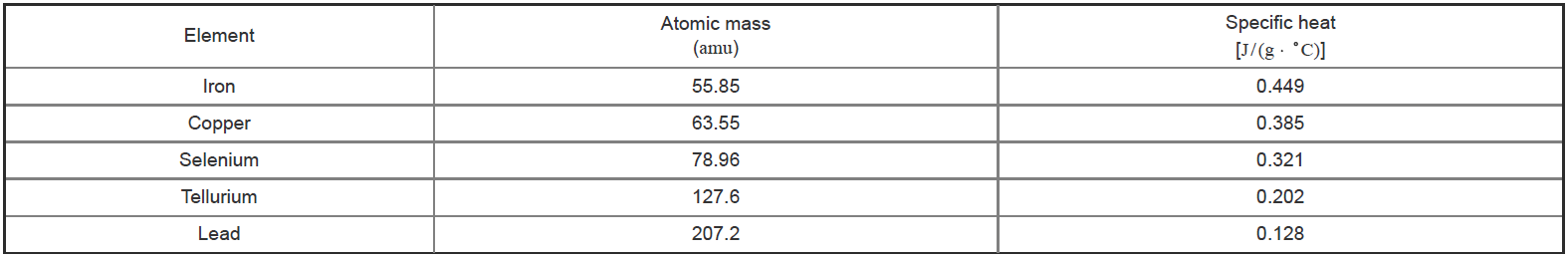Problem: In slope-intercept form, what is the equation for the line in Part B (specific heat vs. atomic mass–1)?Express your answer in terms of x and appropriate constants to two significant figures.Based on specific heat measurements, Pierre Dulong and Alexis Petit proposed in 1818 that the specific heat of an element is related to its atomic weight (atomic mass). Thus, by measuring the specific heat of a new element, its atomic weight could be readily established. The specific heat and atomic mass for a series of elements are provided for reference.

FREE Expert Solution
90% (400 ratings)Problem Details

In slope-intercept form, what is the equation for the line in Part B (specific heat vs. atomic mass–1)?

Express your answer in terms of x and appropriate constants to two significant figures.

Based on specific heat measurements, Pierre Dulong and Alexis Petit proposed in 1818 that the specific heat of an element is related to its atomic weight (atomic mass). Thus, by measuring the specific heat of a new element, its atomic weight could be readily established. The specific heat and atomic mass for a series of elements are provided for reference.